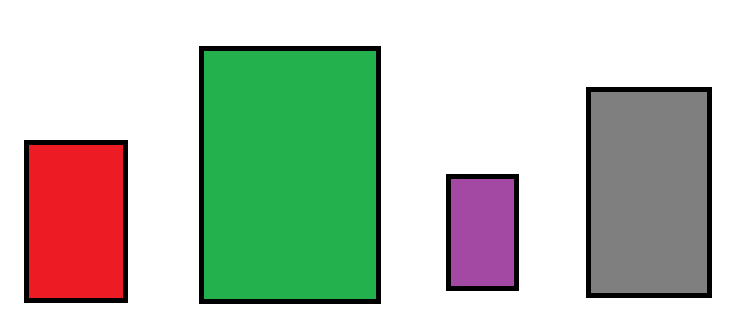﻿ How to Count the Number of Objects in an Image in Python using OpenCV ﻿# How to Count the Number of Objects in an Image in Python using OpenCVIn this article, we show how to count the number of objects in an image in Python using the OpenCV module.

OpenCV is very dynamic in which we can find all the objects (or contours) in an image using the cv2.findContours() function.

In order to find objects in an image, what we are really looking for are external contours. An external contour is the outermost edges of an image. This distinguishes one object from another in an image.

So this is what we will do in this case.

In this example, we will be working with the following image shown below.As you can see in the above image, there are 4 rectangles, which we will say represents containers.

Below we'll see how we can count the number of objects in this image in code.

Let's now go over this code.

First, we import OpenCV using the line, import cv2

Next, we read in the image, which in this case is, Containers.png

We create a grayscale version of the image and then find the Canny edges, which helps simplify the image for cvs.findContours() function. The cv2.findContours() function finds all external contours in our image, which finds the unique objects in an image. We do not want to find internal contours in our image because this is contours that may exist within an object. We are simply looking for external contours only, which means unique objects.

So the variable, contours, holds all the external contours of the image.

To find the number of contours, we use the len() function. This gives us the number of contours (or objects) in the image.

We then print out the number of objects in this image.

So this how we can count the number of objects in an image in Python using OpenCV.

Related Resources